### Introduction to Numerical Analysis: Polynomial Interpolation

### Introduction to Polynomial Interpolation

Polynomial interpolation is the procedure of fitting a polynomial of degreeto a set of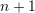data points. For example, if we have two data points, then we can fit a polynomial of degree 1 (i.e., a linear function) between the two points. If we have three data points, then we can fit a polynomial of the second degree (a parabola) that passes through the three points. Figure 1 shows fitting polynomials of degrees 1, 2, 3, and 4, to sets of 2, 3, 4, and 5 data points. The polynomial equation for each curve is shown in the title for each graph.

Polynomial interpolation relies on the fact that for everydata points, there is a unique polynomial of degreethat passes through thesedata points. This fact relies on the Fundamental Theorem of Algebra that states that every degreepolynomial has exactlyroots. So, if two degreepolynomials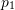and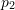agree onpoints, then, their difference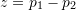is a polynomial of degreebut hasroots which implies that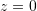.

To find the unique polynomial of degreethat passes throughdata points, we need to solve a linear set ofequations with the coefficients of the polynomial being the unknowns. Let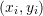be the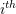data point where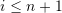. Then, we have: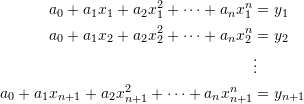Which can be written as follows in matrix form:

(1)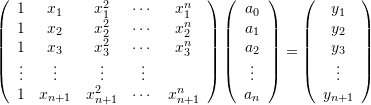The above system can be solved to find the values of the coefficients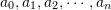which would ensure that the polynomial curve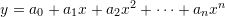passes through thedata points.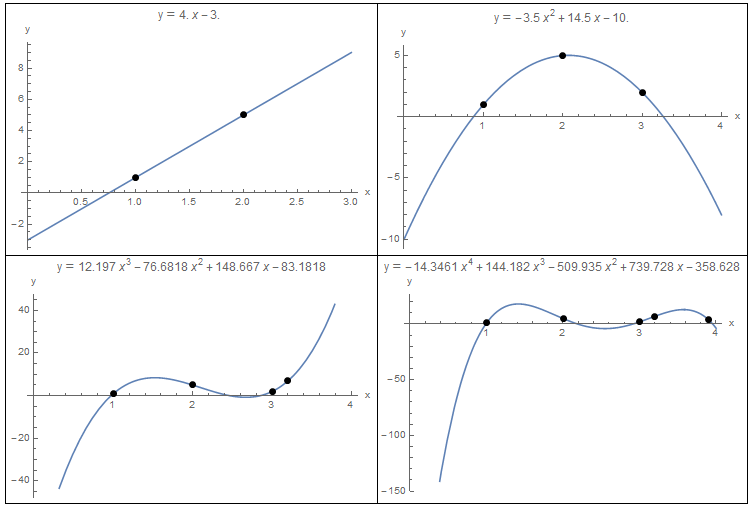Figure 1. Polynomial Interpolation for 2, 3, 4, and 5 data points.

View Mathematica Code
Data = {{1, 1}, {2, 5.}};
y = InterpolatingPolynomial[Data, x];
a = Plot[y, {x, 0, 3}, Epilog -> {PointSize[Large], Point[Data]}, AxesLabel -> {"x", "y"}, ImageSize -> Medium, PlotLabel -> "y" == Expand[y]];

Data = {{1, 1}, {2, 5.}, {3, 2}};
y = InterpolatingPolynomial[Data, x];
b = Plot[y, {x, 0, 4}, Epilog -> {PointSize[Large], Point[Data]},AxesLabel -> {"x", "y"}, ImageSize -> Medium, PlotLabel -> "y" == Expand[y]];

Data = {{1, 1}, {2, 5}, {3, 2}, {3.2, 7}};
y = InterpolatingPolynomial[Data, x];
c = Plot[y, {x, 0, 4}, Epilog -> {PointSize[Large], Point[Data]}, AxesLabel -> {"x", "y"}, ImageSize -> Medium, PlotLabel -> "y" == Expand[y]];

Data = {{1, 1}, {2, 5}, {3, 2}, {3.2, 7}, {3.9, 4}};
y = InterpolatingPolynomial[Data, x];
d = Plot[y, {x, 0, 4}, Epilog -> {PointSize[Large], Point[Data]}, AxesLabel -> {"x", "y"}, ImageSize -> Medium, PlotLabel -> "y" == Expand[y]];
Grid[{{a, b}, {c, d}}, Frame -> All]


#### Example

Find an interpolating polynomial that would fit the data points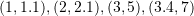.

##### Solution

There are four data points, so, we can fit a third degree polynomial whose coefficients can be found by solving the following system of four linear equations: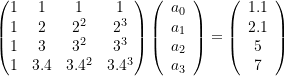Solving the above system yields: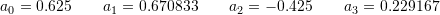Therefore, the interpolating polynomial that would pass through all four data points is: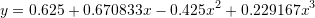The following Mathematica code was used to solve the above equations and produce the plot below:
View Mathematica Code

M = {{1, 1, 1, 1}, {1, 2, 4, 2^3}, {1, 3, 9, 27}, {1, 3.4, 3.4^2, 3.4^3}};
yv = {1.1, 2.1, 5, 7}
a = Inverse[M].yv
y = a[] + a[]*x + a[]*x^2 + a[]*x^3
Plot[y, {x, 0, 4}, Epilog -> {PointSize[Large],  Point[{{1, 1.1}, {2, 2.1}, {3, 5}, {3.4, 7}}]}, AxesLabel -> {"x", "y"}, ImageSize -> Medium, PlotLabel -> "y" == Expand[y]]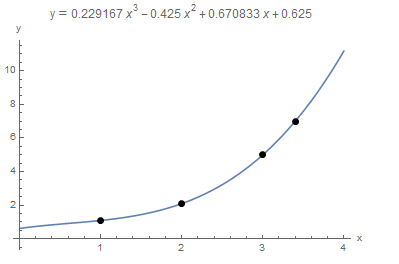Mathematica also has a built-in “InterpolatingPolynomial[Data,x]” function that would automatically form and solve the above equation as shown below. Simply provide the data points as a list and Mathematica would produce the interpolating polynomial as a function of “x”.
View Mathematica Code

Data = {{1, 1.1}, {2, 2.1}, {3, 5}, {3.4, 7}};
y = InterpolatingPolynomial[Data, x];
Plot[y, {x, 0, 4}, Epilog -> {PointSize[Large], Point[Data]}, AxesLabel -> {"x", "y"}, ImageSize -> Medium, PlotLabel -> "y" == Expand[y]]


There are various disadvantages to this interpolating polynomials method. The first disadvantage is that as the number of data points gets bigger, the linear systems of equations that need to be solved become ill-conditioned. In other words, the matrix that needs to be converted would be composed of both small and very large numbers, a situation which is prone to round-off errors. Another major disadvantage is that as the number of data points increases, the order of the interpolating polynomial increases as well. By construction, the polynomial would pass through the data points. However, for large order (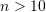), the polynomial would be oscillating wildly between the data points leading to large errors in the interpolated values. The following example illustrates this:

##### Example

Consider the following 11 data points: (-1.00,0.038),(-0.80,0.058),(-0.60,0.100),(-0.4,0.200),(-0.20,0.500),(0.00,1.000),(0.20,0.500),(0.40,0.200),(0.60,0.100),(0.80,0.0580),(1.00,0.038). Using the InterpolatingPolynomial function in Mathematica the 10th order polynomial that would fit through all these points has the form: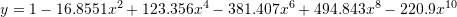From the plot below, the polynomial indeed passes through the 11 points, however, in between the points it oscillates wildly. In fact, for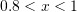the values of the polynomial are much higher than the values at the points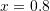and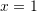which were used for the interpolation: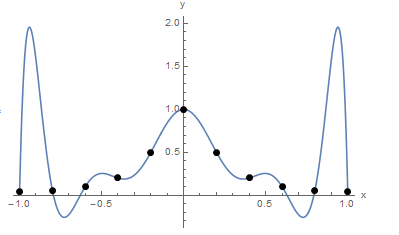It is worth noting that the data points were actually generated using the Runge function: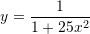When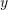is plotted versus the interpolation function, it is clear that the polynomial interpolation function, if used instead ofwould produce erroneous results for values outside of the control points. In fact, Runge used this example in the nineteenth century to show that using higher order polynomials to approximate functions does not always improve accuracy but can have detrimental effects on the values of the function in the intervals between the control points.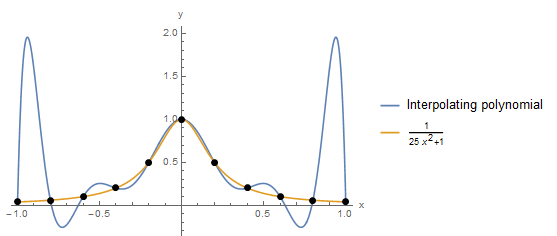View Mathematica Code
Data = {{-1, 0.038}, {-0.8, 0.058}, {-0.60, 0.10}, {-0.4, 0.20}, {-0.2, 0.50}, {0, 1}, {0.2, 0.5}, {0.4, 0.2}, {0.6, 0.1}, {0.8, 0.058}, {1, 0.038}};
y = InterpolatingPolynomial[Data, x]
Chop[Expand[y]]
Plot[y, {x, -1, 1}, Epilog -> {PointSize[Large], Point[Data]}, AxesLabel -> {"x", "y"}, ImageSize -> Medium]
y2 = 1/(1 + 25 x^2)
Plot[{y, y2}, {x, -1, 1}, Epilog -> {PointSize[Large], Point[Data]}, AxesLabel -> {"x", "y"}, ImageSize -> Medium, PlotLegends -> {"Interpolating polynomial", y2}]


### Newton Interpolating Polynomials

As stated above, the matrix formed in Equation 1 can be ill-conditioned and difficult to find an inverse for. A simpler method can be used to find the interpolating polynomial using Newton’s Interpolating Polynomials formula for fitting a polynomial of degreethroughdata pointswith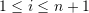: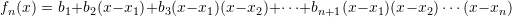where the coefficients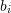are defined recursively using the divided differences as follows: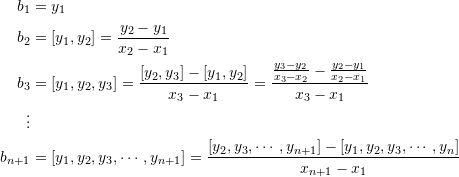This recursive divided differences are illustrated in the following table: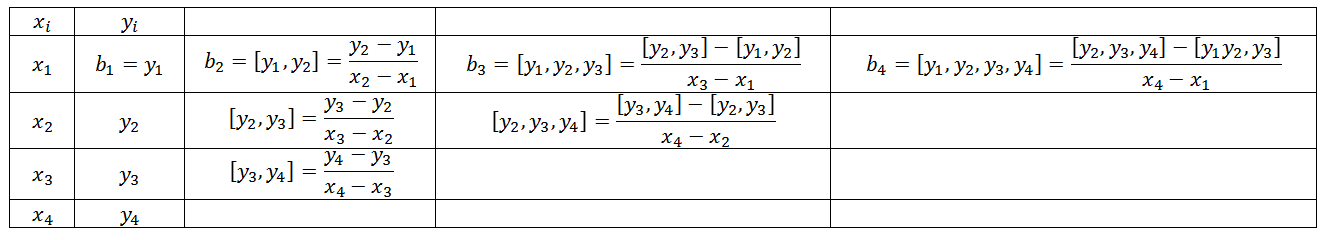The following Mathematica code implements a recursive function “f” that produces the coefficients.
View Mathematica Code

Data = {{1, 3}, {4, 5}, {5, 6}, {6, 8}, {7, 8}};
f[anydata_] := If[Length[anydata] == 1, anydata[[1, 2]], If[Length[anydata] ==2, (anydata[[2, 2]] - anydata[[1, 2]])/(anydata[[2, 1]] -anydata[[1, 1]]), (f[Drop[anydata, 1]] - f[Drop[anydata, -1]])/(anydata[[Length[anydata], 1]] - anydata[[1, 1]])]]
NP[anydata_] := Sum[f[anydata[[1 ;; i]]]*Product[(x - anydata[[j, 1]]), {j, 1, i - 1}], {i, 1, Length[anydata]}]
(*Using the Newton polynomial function*)
y = Expand[NP[Data]]
(*Using the interpolating polynomial built-in function*)
y2 = Expand[InterpolatingPolynomial[Data, x]]


Newton’s formula for generating an interpolating polynomial adopts a form similar to that of a Taylor’s polynomial but is based on finite differences rather than the derivatives. I.e., the coefficientsare calculated using finite difference. One advantage to this form is that the degree of a Newton’s interpolating polynomial can be increased (or decreased) automatically by adding (or removing) more terms corresponding to new points without discarding existing terms.

#### Example

Using Newton’s interpolating polynomials, find the interpolating polynomial to the data: (1,1),(2,5).

##### Solution

We have two data points, so, we will create a polynomial of the first degree. Therefore: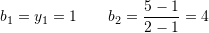Therefore, the interpolating polynomial has the form: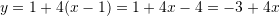#### Example

Using Newton’s interpolating polynomials, find the interpolating polynomial to the data: (1,1),(2,5),(3,2).

##### Solution

We have three data points, so, we will create a polynomial of the second degree. Therefore: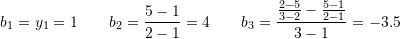Therefore, the interpolating polynomial has the form: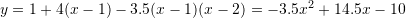#### Example

Using Newton’s interpolating polynomials, find the interpolating polynomial to the data: (1,1),(2,5),(3,2),(3.2,7),(3.9,4).

##### Solution

The divided difference table for these data points were created in excel as follows: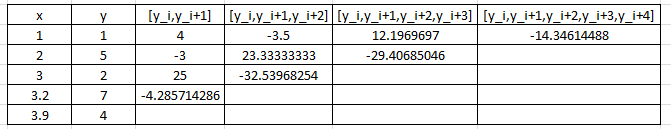Therefore, the Newton’s Interpolating Polynomial has the form: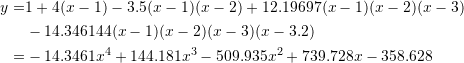### Lagrange Interpolating Polynomials

Another equivalent method to find the interpolating polynomials is using the Lagrange Polynomials. Givendata points: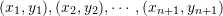, then the Lagrange polynomial of degreethat fits through thedata points has the form: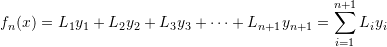where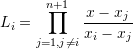In the following Mathematica code a Lagrange polynomial procedure is created to output the Lagrange polynomials.

View Mathematica Code
Data = {{1, 3}, {4, 5}, {5, 6}, {6, 8}, {7, 8}};
Lagrange[anydata_] := Sum[anydata[[i, 2]]*LP[anydata, i], {i, 1, Length[anydata]}]
LP[anydata_, i_] := (newdata = Drop[anydata, {i}]; Product[(x - newdata[[j, 1]])/(anydata[[i, 1]] - newdata[[j, 1]]), {j, 1, Length[newdata]}])
(*Using the Lagrange polynomial function*)
y = Expand[Lagrange[Data]]
(*Using the interpolating polynomial built-in function*)
y2 = Expand[InterpolatingPolynomial[Data, x]]


The Lagrange interpolating polynomials produce the same polynomial as the general method and the Newton’s interpolating polynomials. The examples used for the Newton’s interpolating polynomials will be repeated here.

#### Example

Using Lagrange interpolating polynomials, find the interpolating polynomial to the data: (1,1),(2,5).

##### Solution

We have two data points, so, we will create a polynomial of the first degree. Therefore, the interpolating polynomial has the form: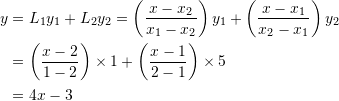#### Example

Using Lagrange interpolating polynomials, find the interpolating polynomial to the data: (1,1),(2,5),(3,2).

##### Solution

We have three data points, so, we will create a polynomial of the second degree. Using Lagrange polynomials: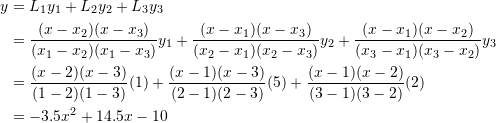#### Example

Using Lagrange polynomials, find the interpolating polynomial to the data: (1,1),(2,5),(3,2),(3.2,7),(3.9,4).

##### Solution

A fourth order polynomial would be needed to pass through five data points. Using Lagrange polynomials, the required function has the form: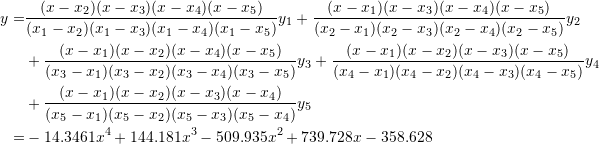### Extrapolation

Extrapolation is the process of predicting a value that lies outside the range of the known base points. Extrapolation using polynomials should be treated with extreme care. For example, the Runge function described above predicts, when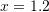, the value of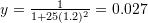. Had we used extrapolation using the fitted polynomial, the predicted value would have been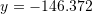which does not really follow the trend of the interpolated points!

### Problems

1. Derive the Newton interpolating polynomial and the Lagrange polynomial formulas.
2. Find 5 examples where an engineer could have some discrete experimental observationsfor specific instances of a variableand would be seeking a relationship to describeversus.
3. The following data points provide the distance in m on a plate and the corresponding measured temperature in Celsius (0,80), (2,53.5), (4,38.43), (6,30.39), (8,30). Find an interpolating polynomial that fits through all the data points. Plot the curve showing the interpolating polynomial and mark the data points on the curve. Use the interpolating polynomial to estimate the temperature at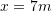.
4. Using the same data given in the previous problem, estimate the temperature atusing linear, quadratic, third, and fourth order Newton polynomial interpolation. For each case, pick enough points for the order of interpolation. Do you get the same answer? Comment on the results.
5. Repeat the previous problem using linear, quadratic, third order, and fourth Lagrange polynomial interpolation. Comment on your answer.
6. The following data provides the average tuition fees in Canadian dollars for engineering students in Alberta from 2010 to 2014 (2010,5401),(2012,5886), (2013,5871), (2014,5929). Use an interpolating polynomial to find an estimate for the tuition fees in 2011. (Data source). Compare your result to the actual value reported by Stats Canada which is: 5815 Canadian dollars.
7. The concentration of a certain toxin in a system of lakes has been monitored at intervals from 1993 to 2003 and was reported as (1993,120), (1995, 127), (1997, 130), (1999, 152), (2001, 182), (2003,198). Using polynomial interpolation, estimate the concentration in 2002. What happens if you remove the first two data points, do you get the same estimate? Comment on your answer.